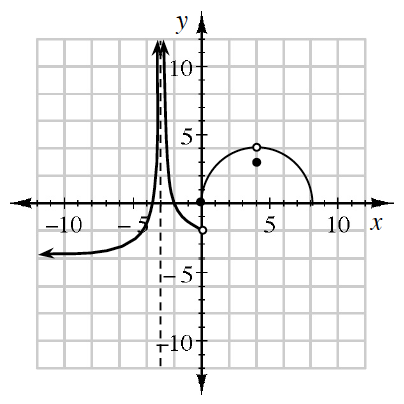### Home > PC3 > Chapter Ch8 > Lesson 8.3.1 > Problem8-95

8-95.

Use the graph at right to evaluate each of the following limits.

1. $\lim\limits_{ x \rightarrow 4 } f ( x )$

Remember to approach on the curve from both sides of $x = 4$.

2. $\lim\limits_ { x \rightarrow 0^-} f ( x )$

Approach on the curve from the left ($-$ side) of $x = 0$ only.

3. $\lim\limits _ { x \rightarrow - \infty } f ( x )$

As $x→∞$, what height does the graph approach?

4. $\lim\limits_ { x \rightarrow 0 } f ( x )$

The function must approach the same height from both sides for the limit to exist.

5. $\lim\limits_ { x \rightarrow - 3 } f ( x )$

What happens at $x = -3$?
Does the limit exist here?